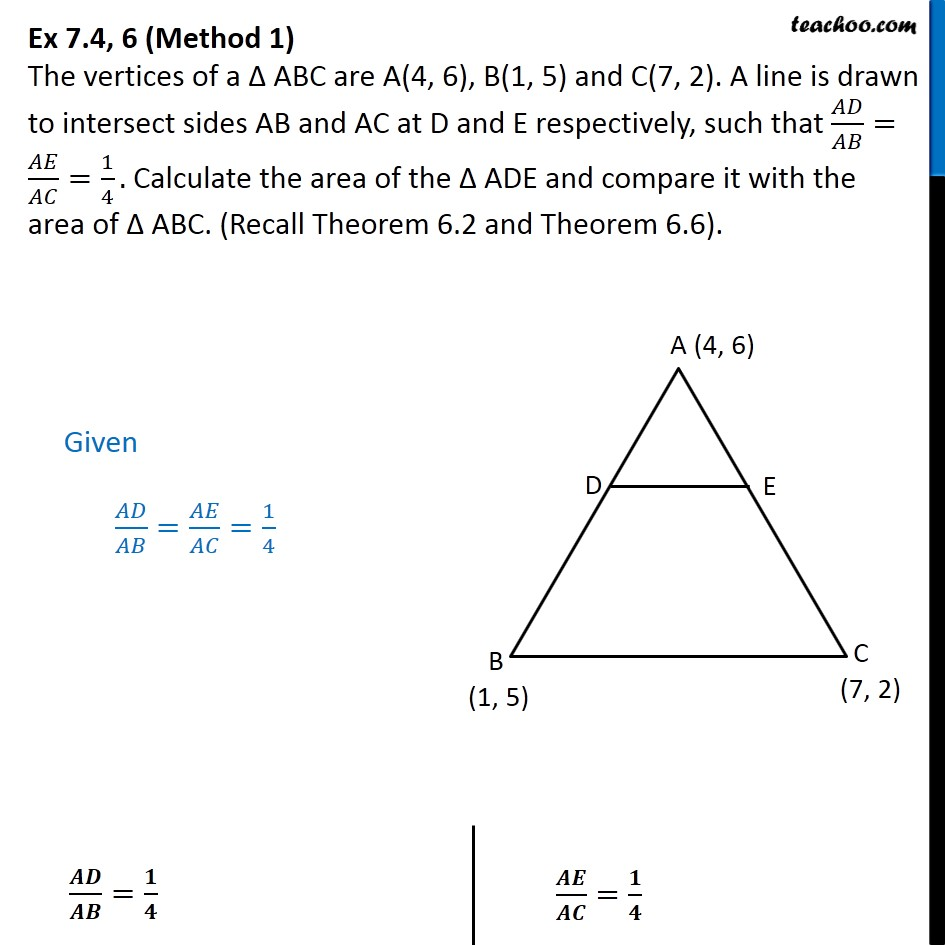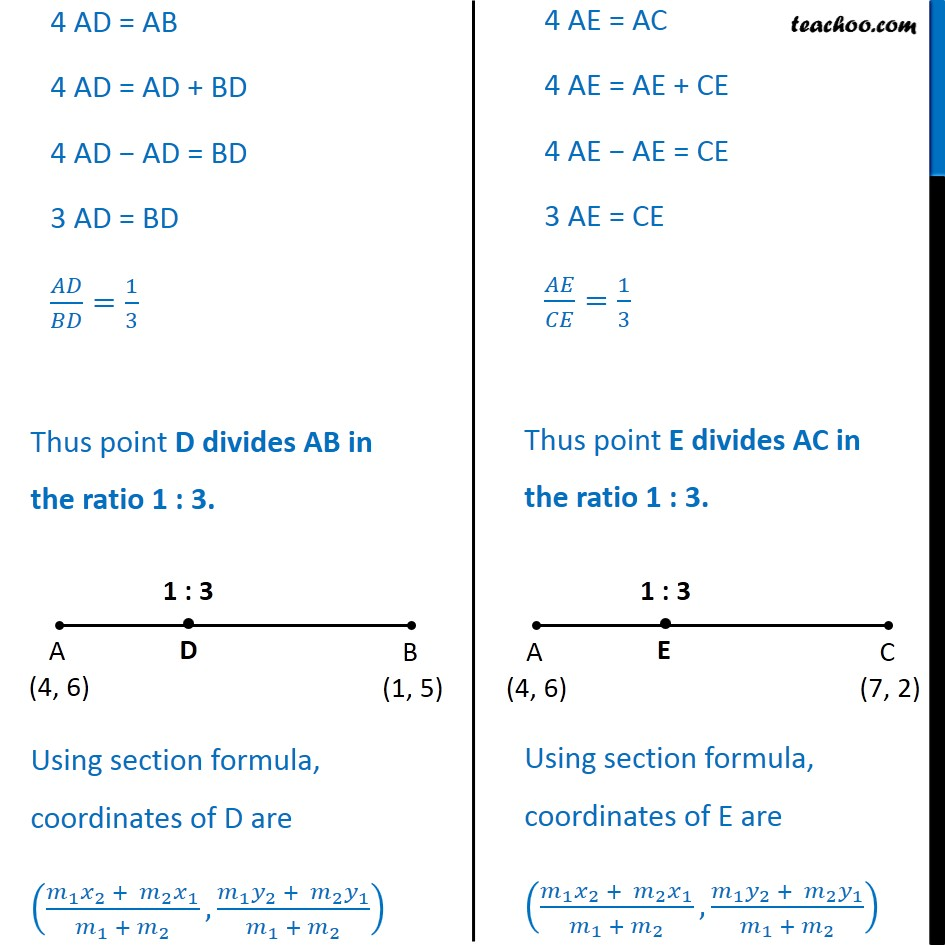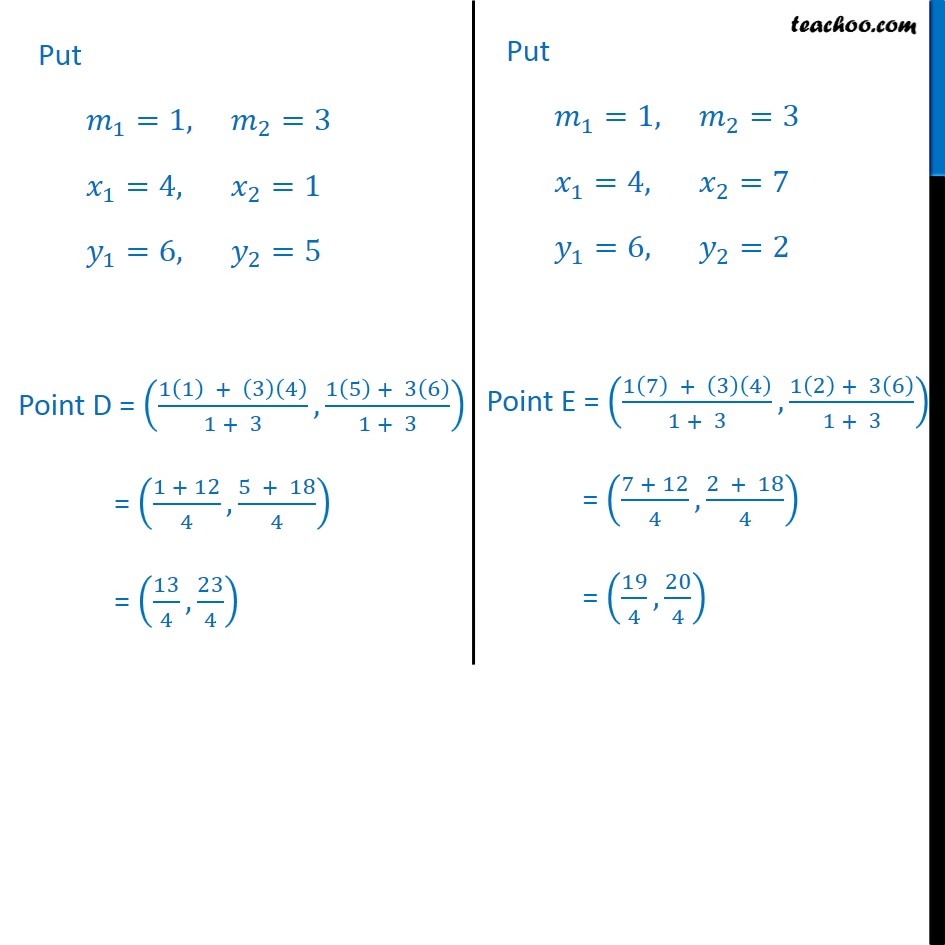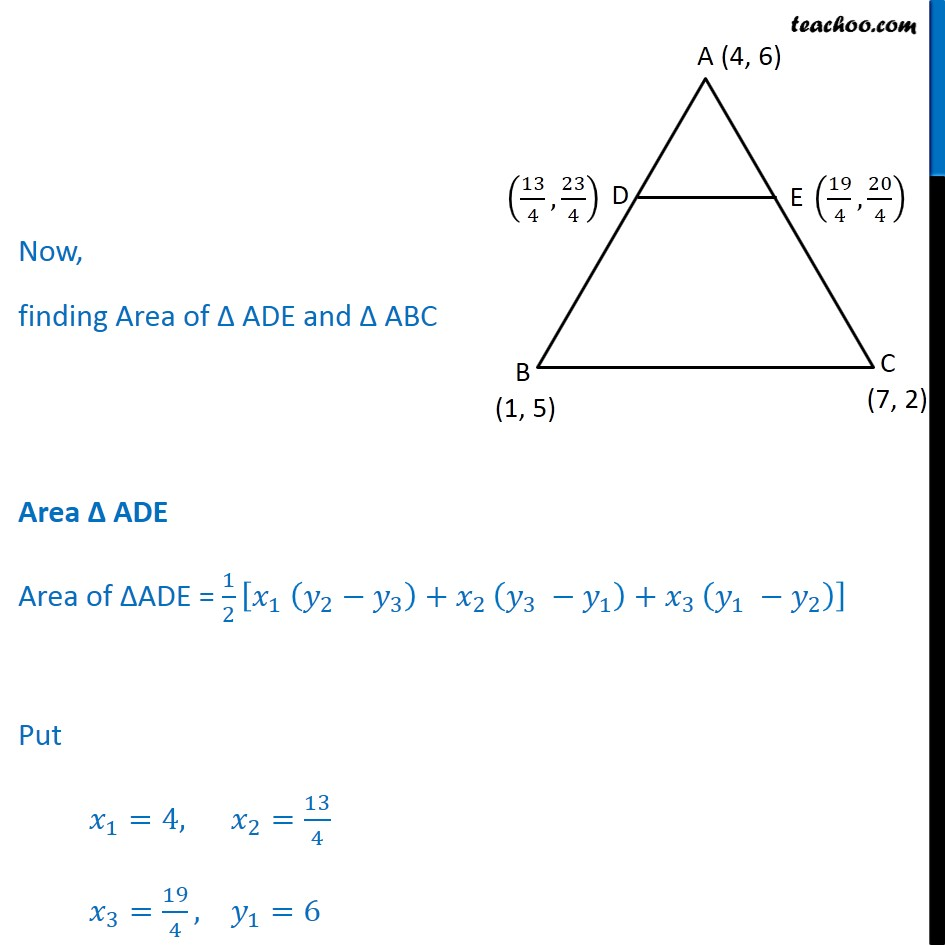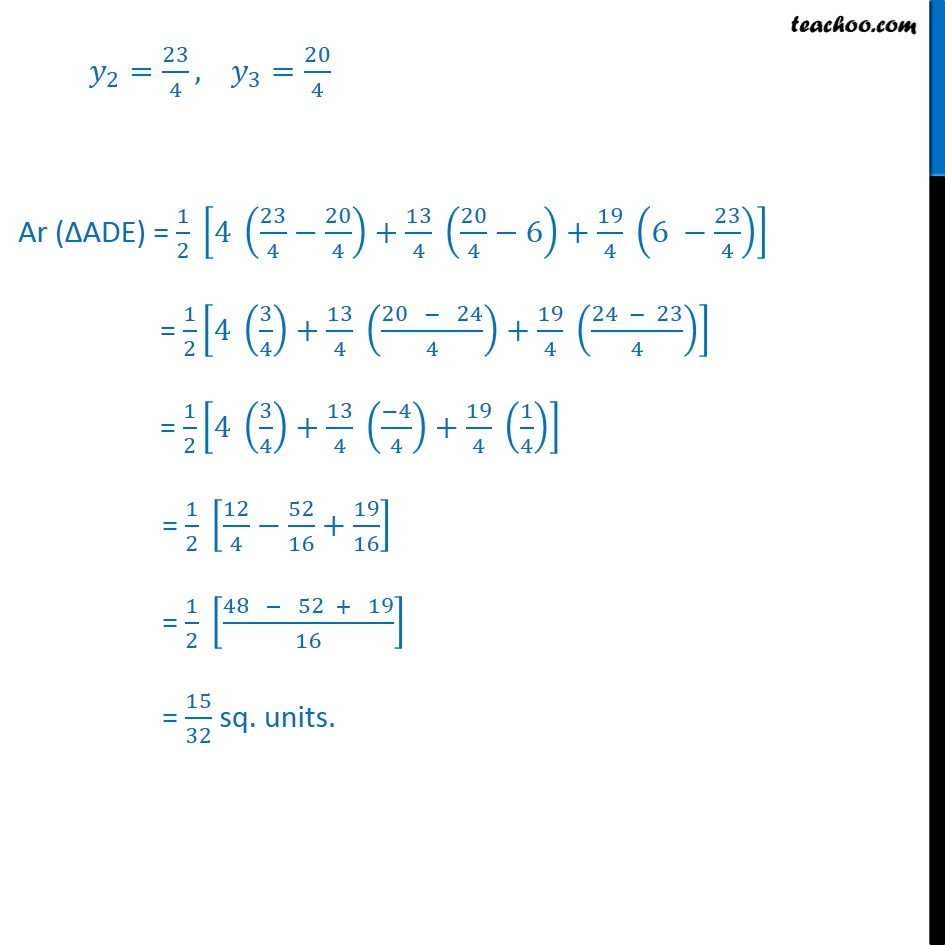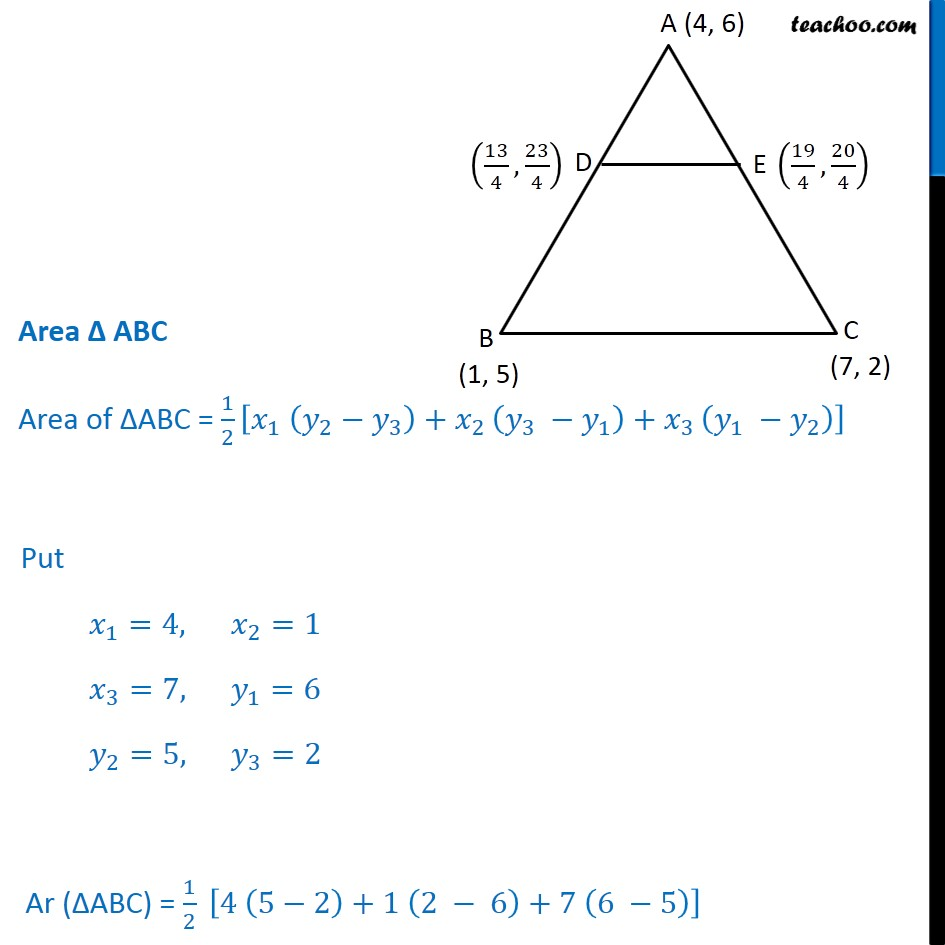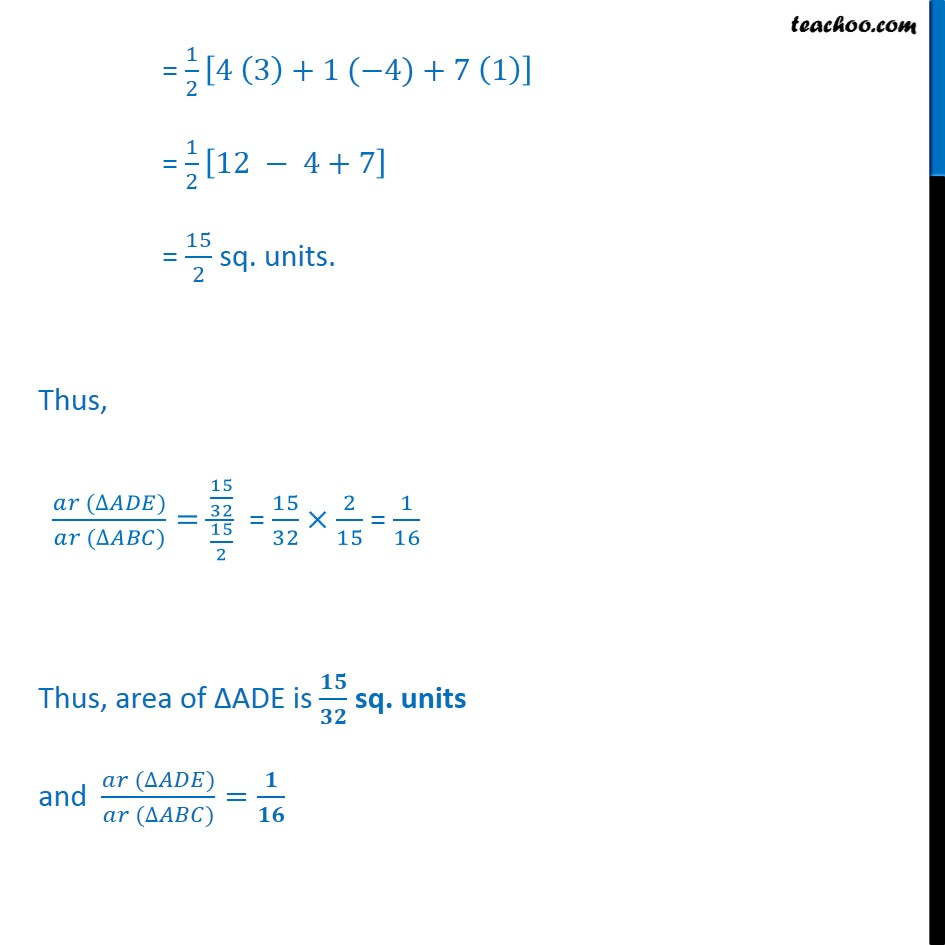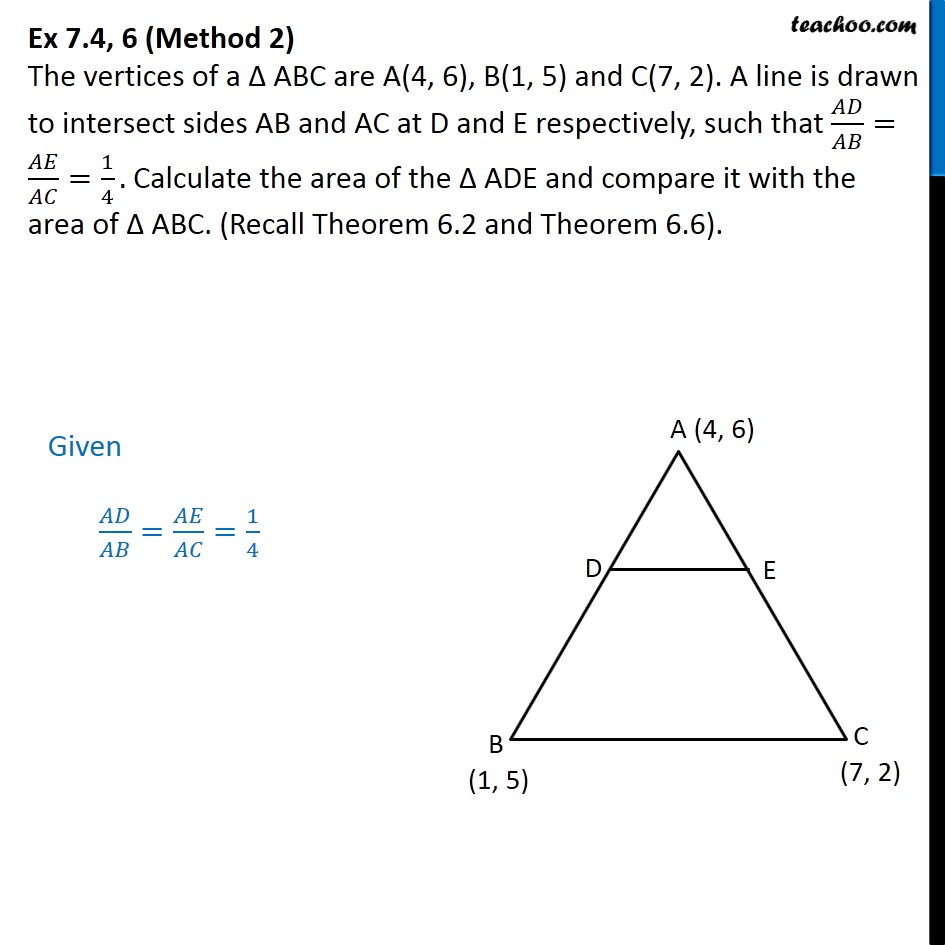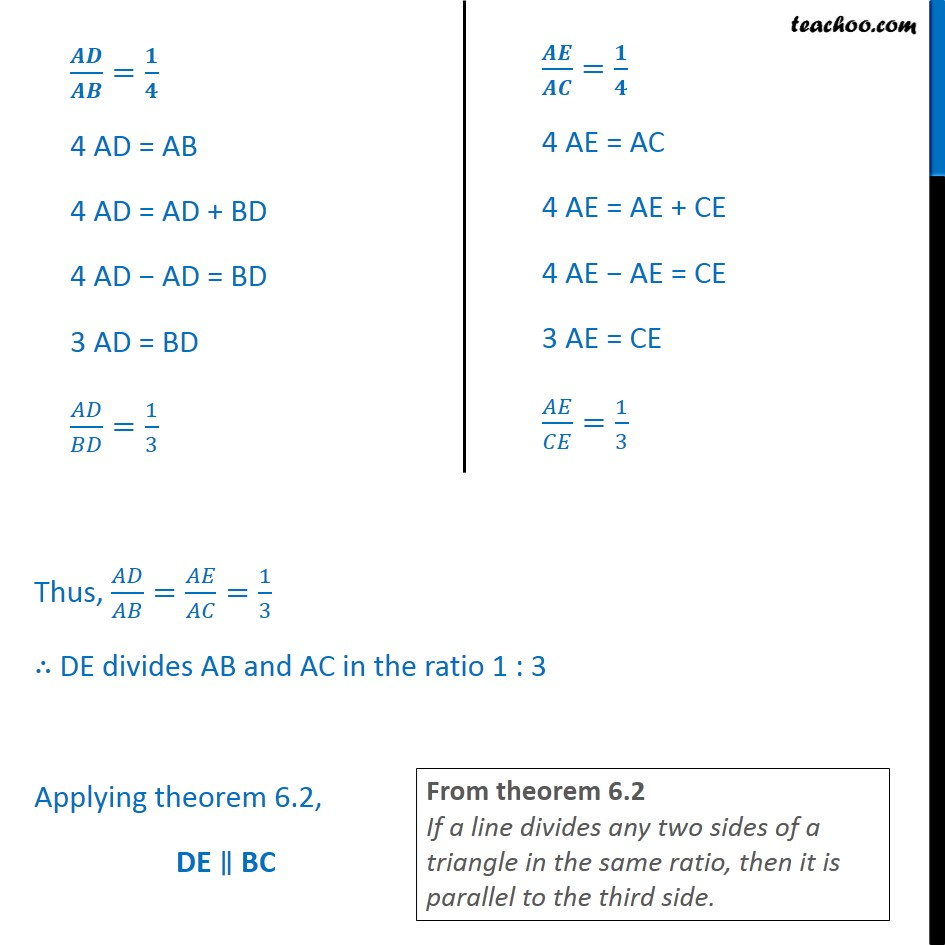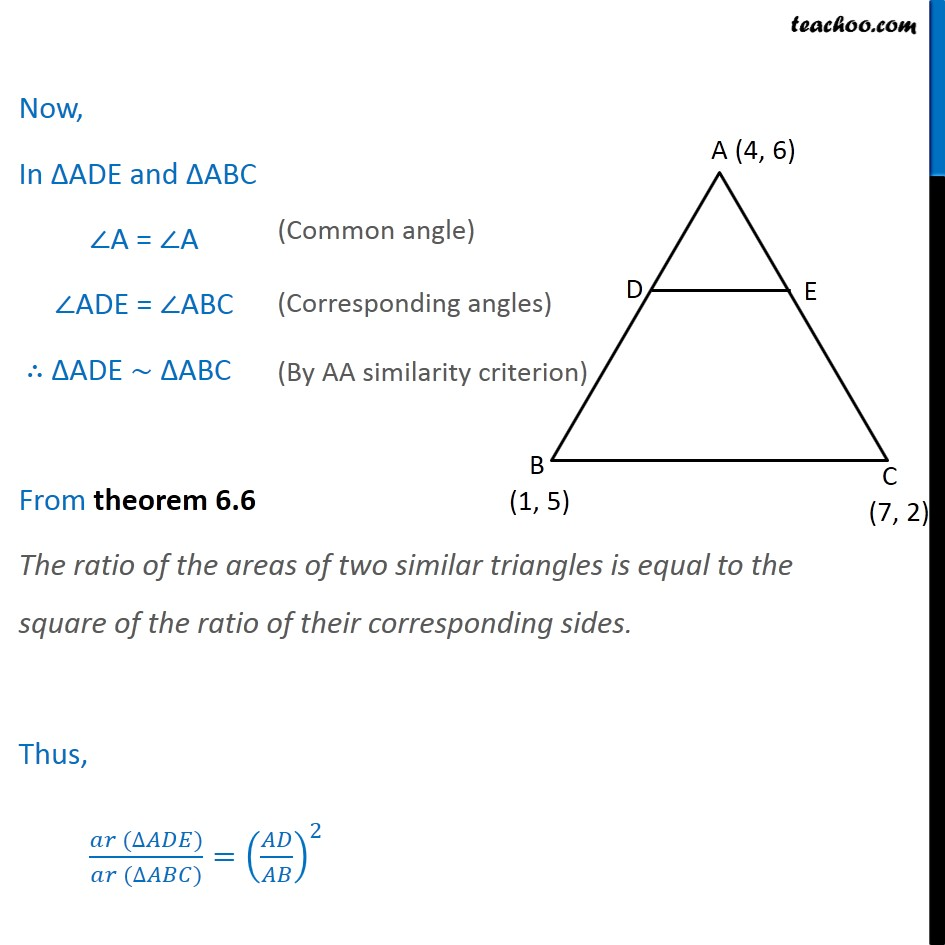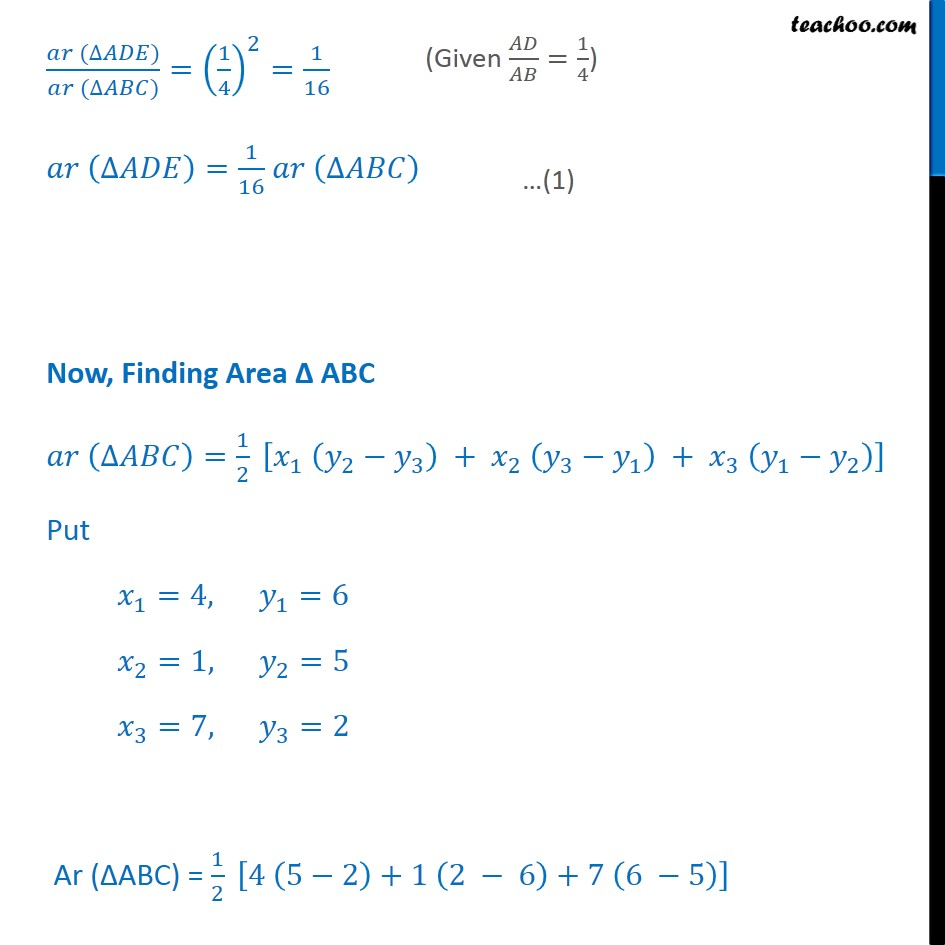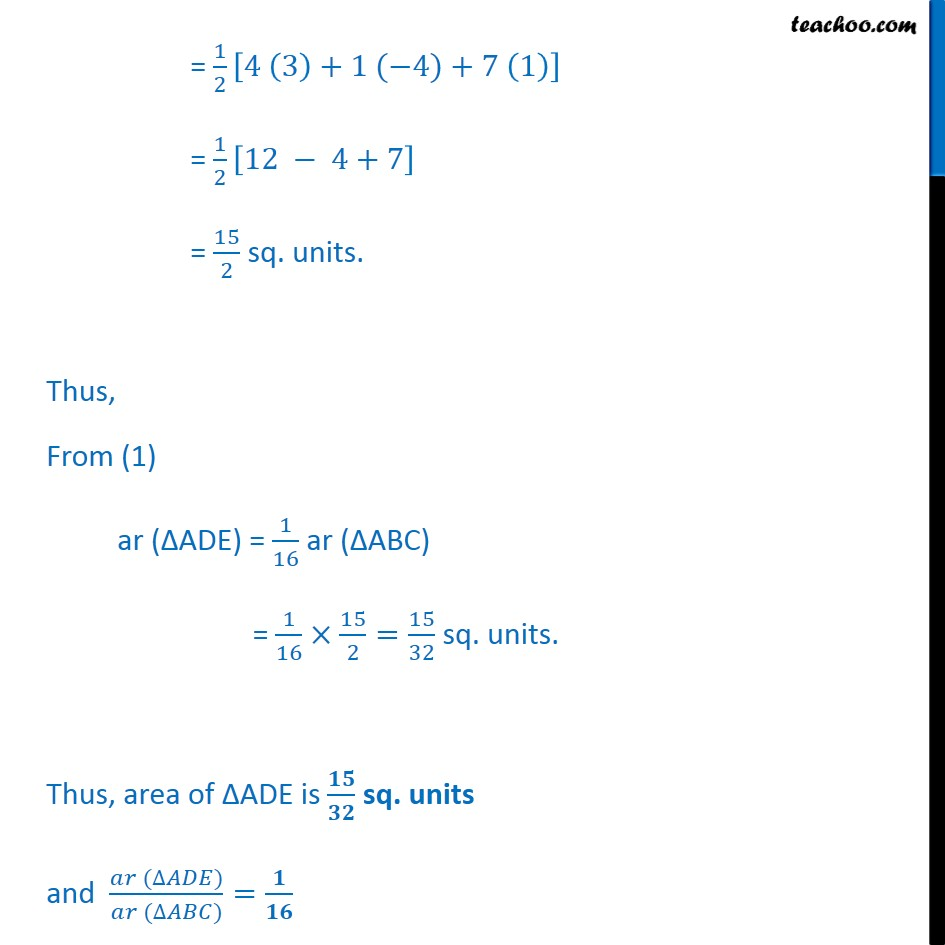1. Chapter 7 Class 10 Coordinate Geometry
2. Serial order wise
3. Ex 7.4 (Optional)

Transcript

Ex 7.4, 6 (Method 1) The vertices of a ABC are A(4, 6), B(1, 5) and C(7, 2). A line is drawn to intersect sides AB and AC at D and E respectively, such that / = / =1/4. Calculate the area of the ADE and compare it with the area of ABC. (Recall Theorem 6.2 and Theorem 6.6). Given / = / =1/4 / = / / = / 4 AD = AB 4 AD = AD + BD 4 AD AD = BD 3 AD = BD / =1/3 Thus point D divides AB in the ratio 1 : 3. Using section formula, coordinates of D are (( _1 _2 + _2 _1)/( _1 + _2 ),( _1 _2 + _2 _1)/( _1 + _2 )) 4 AE = AC 4 AE = AE + CE 4 AE AE = CE 3 AE = CE / =1/3 Thus point E divides AC in the ratio 1 : 3. Using section formula, coordinates of E are (( _1 _2 + _2 _1)/( _1 + _2 ),( _1 _2 + _2 _1)/( _1 + _2 )) Put _1=1, _2=3 _1=4, _2=1 _1=6, _2=5 Point D = ((1(1) + (3)(4))/(1 + 3), (1(5) + 3(6))/(1 + 3)) = ((1 + 12)/4,(5 + 18)/4) = (13/4,23/4) Put _1=1, _2=3 _1=4, _2=7 _1=6, _2=2 Point E = ((1(7) + (3)(4))/(1 + 3), (1(2) + 3(6))/(1 + 3)) = ((7 + 12)/4,(2 + 18)/4) = (19/4,20/4) Now, finding Area of ADE and ABC Area ADE Area of ADE = 1/2 [ _1 ( _2 _3 )+ _(2 ) ( _3 _1 )+ _(3 ) ( _1 _2 )] Put _1=4, _2=13/4 _3=19/4, _1=6 _2=23/4, _3=20/4 Ar ( ADE) = 1/2 [4 (23/4 20/4)+13/4 (20/4 6)+19/4 (6 23/4)] = 1/2 [4 (3/4)+13/4 ((20 24)/4)+19/4 ((24 23)/4)] = 1/2 [4 (3/4)+13/4 (( 4)/4)+19/4 (1/4)] = 1/2 [12/4 52/16+19/16] = 1/2 [(48 52 + 19)/16] = 15/32 sq. units. Area ABC Area of ABC = 1/2 [ _1 ( _2 _3 )+ _(2 ) ( _3 _1 )+ _(3 ) ( _1 _2 )] Put _1=4, _2=1 _3=7, _1=6 _2=5, _3=2 Ar ( ABC) = 1/2 [4 (5 2)+1 (2 6)+7 (6 5)] = 1/2 [4 (3)+1 ( 4)+7 (1)] = 1/2 [12 4+7] = 15/2 sq. units. Thus, ( ( ))/( ( ))=(15/32)/(15/2) = 15/32 2/15 = 1/16 Thus, area of ADE is / sq. units and ( ( ))/( ( ))= / Ex 7.4, 6 (Method 2) The vertices of a ABC are A(4, 6), B(1, 5) and C(7, 2). A line is drawn to intersect sides AB and AC at D and E respectively, such that / = / =1/4. Calculate the area of the ADE and compare it with the area of ABC. (Recall Theorem 6.2 and Theorem 6.6). Given / = / =1/4 / = / 4 AD = AB 4 AD = AD + BD 4 AD AD = BD 3 AD = BD / =1/3 / = / 4 AE = AC 4 AE = AE + CE 4 AE AE = CE 3 AE = CE / =1/3 Thus, / = / =1/3 DE divides AB and AC in the ratio 1 : 3 Applying theorem 6.2, DE BC From theorem 6.2 If a line divides any two sides of a triangle in the same ratio, then it is parallel to the third side. Now, In ADE and ABC A = A ADE = ABC ADE ~ ABC From theorem 6.6 The ratio of the areas of two similar triangles is equal to the square of the ratio of their corresponding sides. Thus, ( ( ))/( ( ))=( / )^2 ( ( ))/( ( ))=(1/4)^2=1/16 ( )=1/16 ( ) Now, Finding Area ABC ( )=1/2 [ _1 ( _2 _3 ) + _2 ( _3 _1 ) + _3 ( _1 _2 )] Put _1=4, _1=6 _2=1, _2=5 _3=7, _3=2 Ar ( ABC) = 1/2 [4 (5 2)+1 (2 6)+7 (6 5)] = 1/2 [4 (3)+1 ( 4)+7 (1)] = 1/2 [12 4+7] = 15/2 sq. units. Thus, From (1) ar ( ADE) = 1/16 ar ( ABC) = 1/16 15/2=15/32 sq. units. Thus, area of ADE is / sq. units and ( ( ))/( ( ))= /

Ex 7.4 (Optional)

About the AuthorDavneet Singh
Davneet Singh is a graduate from Indian Institute of Technology, Kanpur. He has been teaching from the past 9 years. He provides courses for Maths and Science at Teachoo.